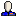The second of my Graph Drawing papers, Trees with Convex Faces and Optimal Angles (withchouyu_31), is now up at arXiv. We draw trees so that, if all leaf edges are extended to infinite rays, the drawing would partition the plane into infinite convex cells; if this is the case, then the edges can't cross no matter what lengths are chosen for them. We show how to find the drawing of this type that maximizes the sharpest angle in the drawing, in linear time for both embedded and free trees; this involves some case analysis for special families of trees in which the angle is obtuse, together with a more general algorithm for trees where the angle is acute. Below is one of the more complex of the obtuse cases, with the edge lengths chosen all equal; we also investigated different algorithms for setting edge lengths once the slopes are chosen.